# C166 – Meter Factor Computation – Master Meter (Mass)

## Description

This calculates the K-factor and the meter factor of a mass based meter using a master meter for proving.

IP Petroleum Measurement Manual: Part X – Meter Proving

Kelton calculation reference C166

KIMS calculation reference K175

## Options

### Factor Type

• K-Factor
• Meter Factor

This option is chosen to…

### Ctsp

• None
• User entered
• Calculated – linear expansion coefficient
• Calculated – cubic expansion coefficient

• None
• User entered
• Calculated

### Ctsm

• None
• User entered
• Calculated – linear expansion coefficient
• Calculated – cubic expansion coefficient

• None
• User entered
• Calculated

## Calculation

### Uncorrected K-factor

The uncorrected K-factor is calculated by: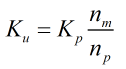Where Kp = Master meter K-factor np = Number of pulses counted by master meter nm = Number of pulses counted by duty meter

### Temperature Correction

The correction factor due to thermal expansion of the steel (Ctsp and Ctsm) is calculated by: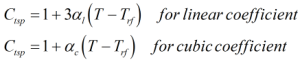Where αc = Cubic temperature coefficient αl = Linear temperature coefficient T = Temperature Trf = Reference temperature

### Pressure Correction

The correction factor due to pressure on the steel (Cpsp and Cpsm) is calculated by: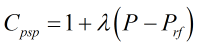λ = Pressure coefficient P = Pressure Prf = Reference pressure

### K-factor

The K-factor is calculated by: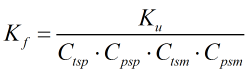Where Ku = Uncorrected K-factor Ctsp = Correction factor for the thermal expansion of the steel of the master meter Cpsp = Correction factor for the expansion of the steel of the master meter due to pressure Ctsm = Correction factor for the thermal expansion of the steel of the meter Cpsm = Correction factor for the expansion of the steel of the meter due to pressure

### Meter Factor

The meter factor is calculated by: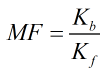Where Kf = Meter K-factor Kb = Base K-factor

Back